Restraint Length Calculator

•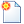New
•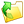Open
•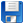Save
•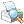Print
•Spreadsheet
Notes
Notes
Pipe Material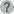Soil TypeSafety FactorTrench TypeDepth of Bury (ft.)Test PressureFitting TypeNominal SizeBend AngleNominal SizeBend AngleLow Side Depth(ft.)Nominal SizeBend AngleLow Side Depth(ft.)Nominal SizeBranch SizeLength Along RunNominal SizeReduced SizeNominal Size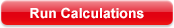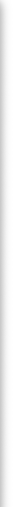Calculated Restraint Length
*all joints within the calculated length must be restrained
*If your distance between fittings is less than or equal to the calculated
restraint length, restrain all joints between those fittings.